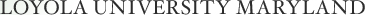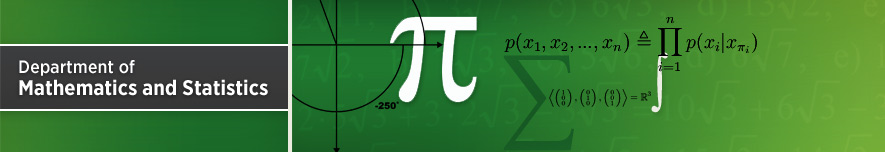## Dr. Kevin Woods

### Room: B01 Knott Hall

Abstract:

We'll start with the following question: "Given two denominations of stamps, a cents and b cents, what is the largest postal rate that we cannot pay exactly?" This is called the Frobenius problem with two generators. Using generating functions, we'll get a nice formula for the answer.

Then we'll take a look at a wide variety of questions that we can answer using this same sort of generating function. In general, generating functions can often encode a seemingly complicated set (such as the set of postal rates that can be paid with a cent and b cent stamps) in a nice, compact form. Then we can use the generating functions to answer questions like "Is this set nonempty?" "What is its cardinality?" "What is its maximal element?" We'll build up a theory of what can be done with generating functions, and we will approach these problems from an algorithmic perspective: what can we do "quickly" (that is, in polynomial time)?

Back to Current Seminars page